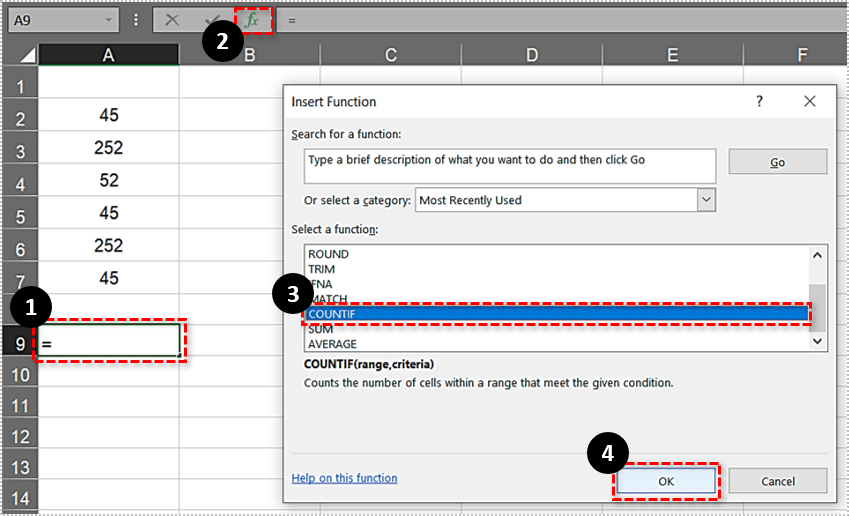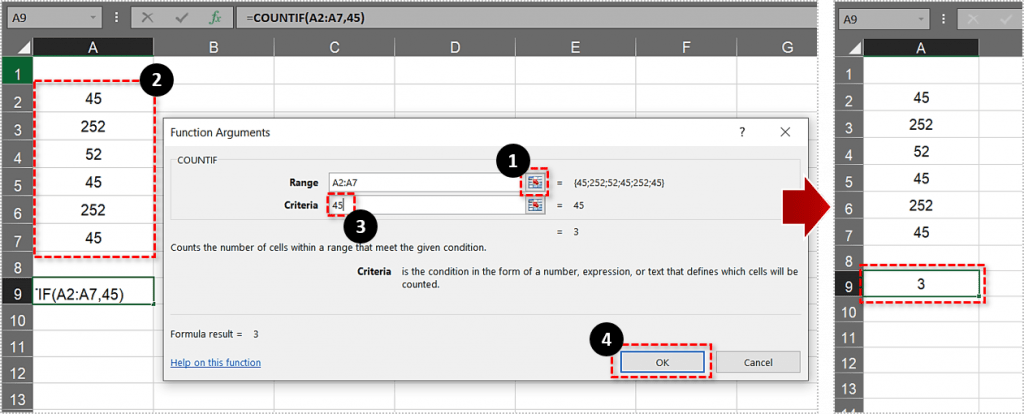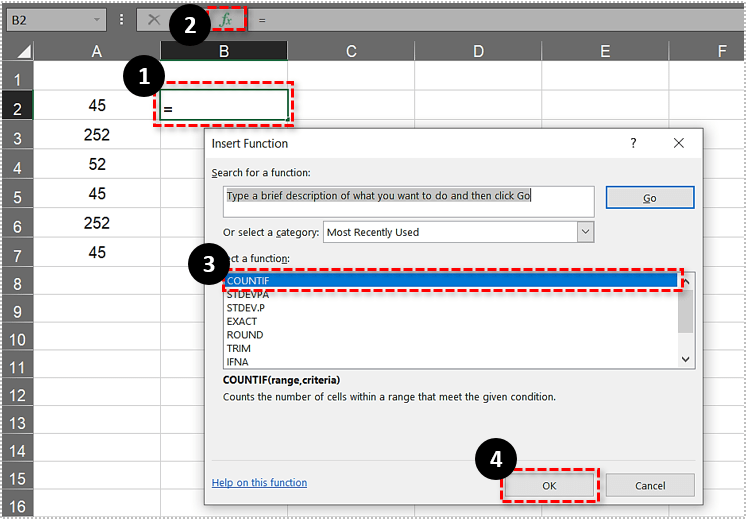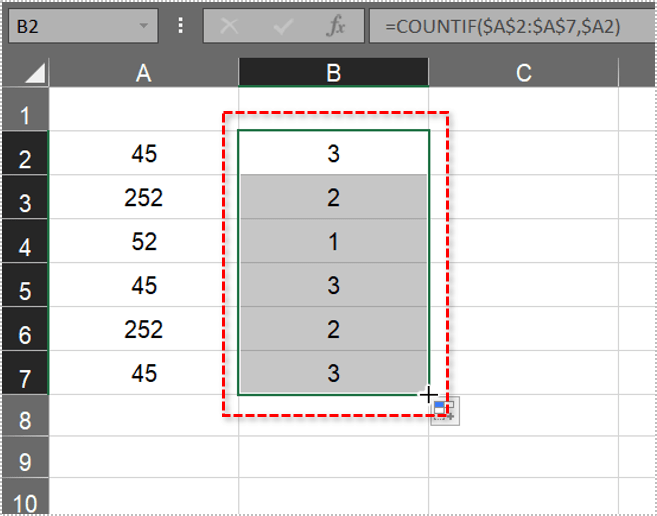# How To Count Duplicates in Excel Spreadsheets

Spreadsheets are one of the most powerful and easy-to-use ways to organize, view, and manipulate all sorts of data. One of the most common tasks people perform using spreadsheets like Microsoft Excel is to analyze data sets. Often, a spreadsheet may include duplicated data, that is, a row or cell that duplicates another row or cell. Sometimes we want to remove those duplicates, and we created an article on removing duplicates in Excel to teach how to do that. However, sometimes we don’t want to change the data, but we do want to know, for example, how many times a particular value is duplicated in our data set. This article will teach you several different ways to count duplicates in Excel spreadsheets.

## The COUNTIF Function

COUNTIF is undoubtedly one of the more powerful and convenient Excel statistical functions. COUNTIF works by finding the total number of cells within a selected range which match a specified criteria. For example, you can ask COUNTIF to tell you how many cells in column D contain the phrase “Excel is groovy”. The syntax for this Excel function is: =COUNTIF (range, criteria). The range is the cells in which you want to search for the criteria, criteria is what you want the function to count. So how do we use COUNTIF to count duplicate values?

First, enter some dummy data into a blank Excel spreadsheet. Enter the values ’45,’ ‘252,’ ’52,’ ’45, ‘252’ and ’45’ into the cells A2:A7. Then your spreadsheet should match the one shown directly below.

Now let’s suppose you need to find how many cells include the duplicate value 45. The COUNTIF function will tell you that in a jiffy!

1. Select cell A9, and then press the fx buton.
2. Select COUNTIF and press OK to open the window shown directly below.(If you are comfortable with Excel formulas, you can just type the formula directly into the cell without using the dialog boxes)3. Click the Range button and select the cell range A2:A7.
4. Next, enter 45 in the Criteria text box and press OK to close the window.A9 will now return the formula result of 3. Thus, there are three cells within the selected range that include the value 45.

The function works much the same for text. As an example, enter ‘pear,’ ‘apple,’ ‘apple’ and ‘orange’ in cells A11:14. Then the spreadsheet should include a short list of fruit as shown directly below.

1. Select to add the COUNTIF function to cell A16.
2. Press the fx button, select COUNTIF and click OK.

Now when you press OK, A16 should return the value 2. So there are two cells that include apple duplicates. Note that cells within the selected range must not contain any spaces. If they do, Excel won’t count them as duplicates (unless the criteria entered also includes exactly the same empty spaces). This Tech Junkie guide tells you about how to remove empty spaces from Excel spreadsheet cells.

## Count Multiple Duplicate Values

But what if you needed to find the total number of duplicates for two, three or more values? For example, you might need to find how many times three sets of values are duplicated within a cell range. In which case, you can expand the COUNTIF function so that it includes multiple criteria.

2. Then click in the fx bar to edit the original function. Add +COUNTIF(A2:A7,252) to the function, and press Enter.

The full function will then effectively become =COUNTIF(A2:A7,45)+COUNTIF(A2:A7,252) as shown in the snapshot directly below. A9 will then return the value 5. As such, the function has totaled both the 45 and 252 duplicates within our cell range, which amounts to 5.

The function can also count values in cell ranges across multiple Excel spreadsheets. That would require modifying the required cell ranges so that they include a sheet reference, such as Sheet2! Or Sheet3!, in the cell reference. For example, to include a range of cells in Sheet 3, the function would be something like: =COUNTIF(A2:A7,45)+COUNTIF(Sheet3!C3:C8,252).

## Count All Duplicate Values Within a Column or Row

Some Excel users might need to count all the duplicate values or items within a spreadsheet column. You can also do that with the COUNTIF function. However, the function requires an absolute cell reference for the entire column you need to count all the duplicates in.

2. Click the fx button and select to open the COUNTIF Function Arguments window.3. Enter \$A\$2:\$A\$7 in the Range box.
4. Input \$A2 in the Criteria box and press OK to add the function to the spreadsheet.5. Cell B2 will return the value 3 as shown below.
6. Now you need to copy the function to all the cells below it down to B7. Select B2 then left click the bottom-right corner and drag it down to B7.That copies the function to all the other cells as shown directly below.

Column B in the shot above now effectively counts all the values within the range A2:A7. It highlights that 45 duplicates three times and that 252 duplicates twice within the selected range. So now you can quickly find all repeated values in spreadsheet columns or rows by including absolute cell references within the COUNTIF function.

Now you can count any number of duplicate values or items on your Excel spreadsheets with COUNTIF. Open this YouTube page to see the COUNTIF function in action.

Know any other cool Excel tips and techniques? Share them with us below!

## 2 thoughts on “How To Count Duplicates in Excel Spreadsheets”Mark says:
Thanks worked a treat, one question though, how would you make the formula count the number of visible rows in a spreadsheet. i.e. one that has been filtered by date.
For example 3 years of data and the formula tells me a number has occurred 42 times in three years. When I change the date filter to only 2018 the number of duplicates does not change as it still counting the invisible rowsKamila says:
That would be COUNTIFS. You have to include the year column as one of the ranges instead of filtering it and setting the specific year which you want countedAli says:
How I could count if I have many number, let say I have 2000 rows and I have different numbers for car plats and I want to account how many cars by the record I have.